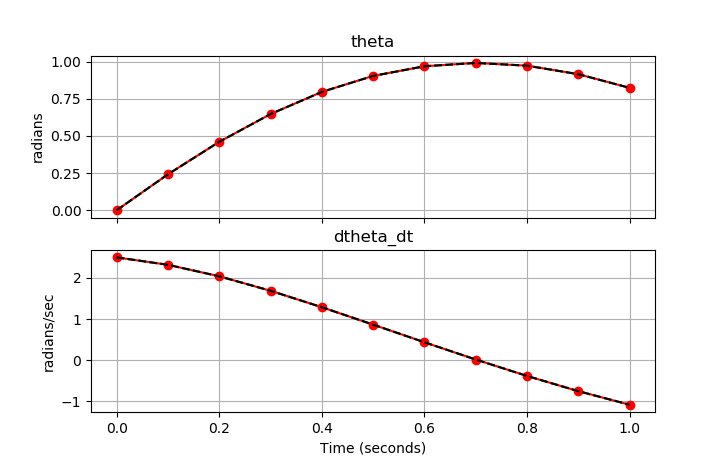## Main.Simulation History

March 19, 2019, at 08:36 PM by 10.37.9.255 -

Three simulations show simultaneous simulation (IMODE=4), sequential simulation (IMODE=7), and simultaneous simulation in a Python loop (IMODE=4).

March 19, 2019, at 08:34 PM by 10.37.9.255 -
Changed lines 21-103 from:

Dynamic simulation is the easiest dynamic mode to configure and run. The requirement for a square problem facilitates model convergence as the solver has only to achieve feasibility with the equality constraints.

to:

Dynamic simulation is the easiest dynamic mode to configure and run. The requirement for a square problem facilitates model convergence as the solver has only to achieve feasibility with the equality constraints.

Example Code (Python GEKKO) with IMODE 4 and 7(:source lang=python:) from gekko import GEKKO import numpy as np import matplotlib.pyplot as plt

1. Number of timesteps

nt = 11 tm = np.linspace(0, 1, nt)

1. Initialize GEKKO

p1 = GEKKO() p2 = GEKKO() p3 = GEKKO()

1. define model

for p in [p1,p2,p3]:

    if p==p3:
p.time = [tm,tm]
else:
p.time = tm

# Model parameters
p.g = p.Const(value=9.81, name='g')
p.l = p.Const(value=2., name='length')
p.m = p.Const(value=1.0, name='mass')
p.f = p.Const(value=0.5, name='friction coefficient')

# State Variables
p.theta = p.Var(value=0, name='angle')
p.dtheta_dt = p.Var(value=2.5, name='angular velocity')

# Equations
p.Equation(p.theta.dt() == p.dtheta_dt)
p.Equation(p.dtheta_dt.dt() ==                -p.g/p.l*p.sin(p.theta) - p.f/p.m*p.dtheta_dt)
p.options.NODES=5

1. Solve simultaneously

p1.options.IMODE=4 p1.solve(disp=False)

p2.options.IMODE=7 p2.solve(disp=False)

p3.options.IMODE=4 th = np.ones_like(tm) dth = np.ones_like(tm) th = 0 dth = 2.5 import time for i in range(1,nt):

    p3.solve(disp=False)

# record values for plotting
th[i] = p3.theta.value
dth[i] = p3.dtheta_dt.value

1. Plot results

fig, axes = plt.subplots(2, 1, sharex=True, figsize=(8,7))

axes.plot(tm, p1.theta.value, 'o-',color='red') axes.plot(tm, p2.theta.value, ':',color='green') axes.plot(tm, th, ,color='black') axes.set_title("theta") axes.set_ylabel('radians') axes.grid()

axes.plot(tm, p1.dtheta_dt.value, 'o-',color='red') axes.plot(tm, p2.dtheta_dt.value, ':',color='green') axes.plot(tm, dth, ,color='black') axes.set_title("dtheta_dt") axes.set_ylabel('radians/sec') axes.grid() axes.set_xlabel('Time (seconds)')

plt.show() (:sourceend:)

June 09, 2017, at 12:59 AM by 10.5.113.159 -
Changed lines 5-7 from:
 nlc.imode = 4 (simultaneous dynamic simulation)
nlc.imode = 7 (sequential dynamic simulation)

to:
 apm.imode = 4 (simultaneous dynamic simulation)
apm.imode = 7 (sequential dynamic simulation)

Changed lines 9-10 from:
 apm_option(server,app,'nlc.imode',7);

to:
 apm_option(server,app,'apm.imode',7);

Changed line 12 from:
 apm_option(server,app,'nlc.imode',4)

to:
 apm_option(server,app,'apm.imode',4)

June 16, 2015, at 06:59 PM by 45.56.3.184 -
Changed lines 5-7 from:
 NLC.imode = 4
or
NLC.imode = 7

to:
 nlc.imode = 4 (simultaneous dynamic simulation)
nlc.imode = 7 (sequential dynamic simulation)

% MATLAB example
apm_option(server,app,'nlc.imode',7);

# Python example
apm_option(server,app,'nlc.imode',4)

June 16, 2015, at 03:15 PM by 45.56.3.184 -
Changed lines 3-5 from:

The DBS file parameter imode is used to control the simulation mode. This option is set to 4 for dynamic simulation.

NLC.imode = 4

to:

The DBS file parameter imode is used to control the simulation mode. This option is set to 4 (simultaneous simulation) or 7 (sequential simulation) for dynamic simulation.

 NLC.imode = 4
or
NLC.imode = 7

October 02, 2008, at 08:57 PM by 158.35.225.228 -
Changed lines 5-14 from:

NLC.imode = 4

to:

NLC.imode = 4

Like steady-state simulation, dynamic simulation requires a square problem with no degrees of freedom (neqn=nvar). Dynamic simulation has many useful purposes including

• Investigate step response characteristics of a nonlinear model
• Simulate process changes for design, trouble-shooting, or planning
• Perform what-if scenarios
• Simulate a virtual process

Dynamic simulation is the easiest dynamic mode to configure and run. The requirement for a square problem facilitates model convergence as the solver has only to achieve feasibility with the equality constraints.

September 30, 2008, at 03:18 PM by 158.35.225.227 -